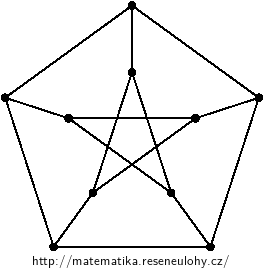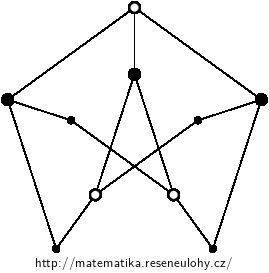## Petersen graph is not planar

Prove in two ways that the Petersen graph is not planar.• #### Solution

The first way: by using the Kuratowski’s theorem, because it contains a subdivision of $$K_ {3{,}3}$$The second way: the contractions maintain planarity, but by the contraction of the matching between the outer and inner five-cycle we get a non-planar graph $$K_5$$.

Third way: Petersen’s graph has no triangles or fourcycles. If it was planar, it should, as a consequence of Euler’s formula, satisfy the estimate $$| E_P | \le \frac53 (| V_P | -2)$$, which it does not satisfy.